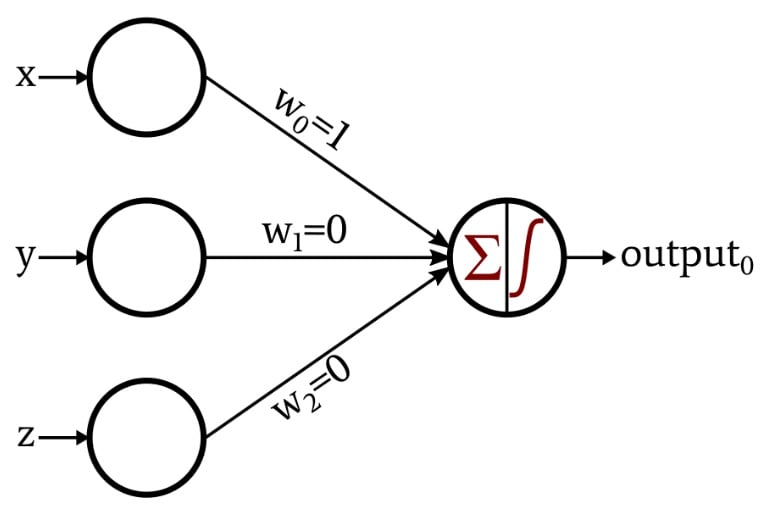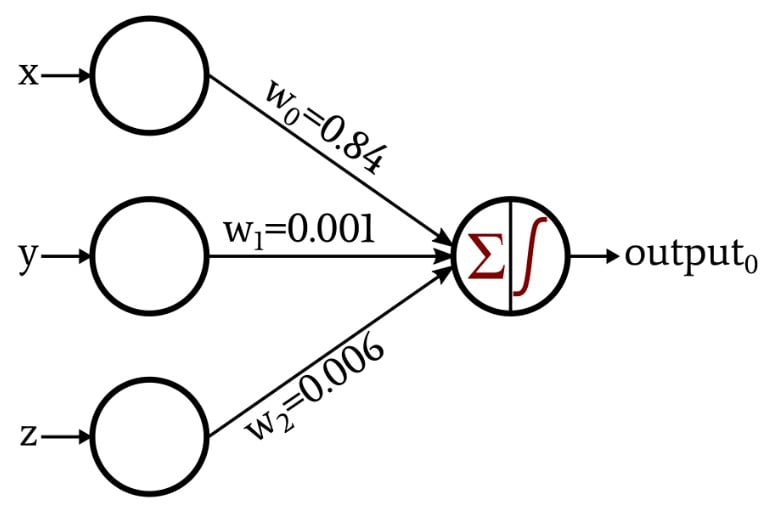Technical Article

# How to Train a Basic Perceptron Neural Network

November 24, 2019 by Robert Keim

## This article presents Python code that allows you to automatically generate weights for a simple neural network.

Welcome to AAC's series on Perceptron neural networks. If you're looking to start from the beginning for background or jump ahead, check out the rest of the articles here:

### Classification with a Single-Layer Perceptron

The previous article introduced a straightforward classification task that we examined from the perspective of neural-network-based signal processing. The mathematical relationship required for this task was so simple that I was able to design the network just by thinking about how a certain set of weights would allow the output node to correctly categorize the input data.

This is the network that I designed:The activation function in the output node is the unit step:

$f(x)=\begin{cases}0 & x < 0\\1 & x \geq 0\end{cases}$

The discussion became a bit more interesting when I presented a network that created its own weights through the procedure known as training:In the rest of this article, we’ll examine the Python code that I used to obtain these weights.

### A Python Neural Network

Here is the code:

import pandas

import numpy as np

input_dim = 3

learning_rate = 0.01

Weights = np.random.rand(input_dim)

#Weights = 0.5

#Weights = 0.5

#Weights = 0.5

Expected_Output = Training_Data.output

Training_Data = Training_Data.drop(['output'], axis=1)

Training_Data = np.asarray(Training_Data)

training_count = len(Training_Data[:,0])

for epoch in range(0,5):

for datum in range(0, training_count):

Output_Sum = np.sum(np.multiply(Training_Data[datum,:], Weights))

if Output_Sum < 0:

Output_Value = 0

else:

Output_Value = 1

error = Expected_Output[datum] - Output_Value

for n in range(0, input_dim):

Weights[n] = Weights[n] + learning_rate*error*Training_Data[datum,n]

print("w_0 = %.3f" %(Weights))

print("w_1 = %.3f" %(Weights))

print("w_2 = %.3f" %(Weights)) 

Let’s take a closer look at these instructions.

### Configuring the Network and Organizing Data

input_dim = 3

The dimensionality is adjustable. Our input data, if you recall, consists of three-dimensional coordinates, so we need three input nodes. This program does not support multiple output nodes, but we’ll incorporate adjustable output dimensionality into a future experiment.

learning_rate = 0.01

We’ll discuss learning rate in a future article.

Weights = np.random.rand(input_dim)

#Weights = 0.5

#Weights = 0.5

#Weights = 0.5

Weights are typically initialized to random values. The numpy random.rand() function generates an array of length input_dim populated with random values distributed over the interval [0, 1). However, the initial weight values influence the final weight values produced by the training procedure, so if you want to evaluate the effects of other variables (such as training-set size or learning rate), you can remove this confounding factor by setting all the weights to a known constant instead of a randomly generated number.

Training_Data = pandas.read_excel("3D_data.xlsx")

I use the pandas library to import training data from an Excel spreadsheet. The next article will go into more detail on the training data.

Expected_Output = Training_Data.output

Training_Data = Training_Data.drop(['output'], axis=1)

The training data set includes input values and corresponding output values. The first instruction separates the output values and stores them in a separate array, and the next instruction removes the output values from the training data set.

Training_Data = np.asarray(Training_Data)

training_count = len(Training_Data[:,0])

I convert the training data set, which is currently a pandas data structure, into a numpy array and then look at the length of one of the columns to determine how many data points are available for training.

### Calculating Output Values

for epoch in range(0,5):

The length of one training session is governed by the number of training data available. However, you can continue optimizing the weights by training the network multiple times using the same data set—the benefits of training don’t disappear simply because the network has already seen these training data. Each complete pass through the entire training set is called an epoch.

for datum in range(0, training_count):

The procedure contained in this loop occurs one time for each row in the training set, where “row” refers to a group of input data values and the corresponding output value (in our case, an input group consists of three numbers representing x, y, and z components of a point in three-dimensional space).

Output_Sum = np.sum(np.multiply(Training_Data[datum,:], Weights))

The output node must sum the values delivered by the three input nodes. My Python implementation does this by first performing an element-wise multiplication of the Training_Data array and the Weights array and then calculating the summation of the elements in the array produced by that multiplication.

if Output_Sum < 0:

Output_Value = 0

else:

Output_Value = 1

An if-else statement applies the unit-step activation function: if the summation is less than zero, the value generated by the output node is 0; if the summation is equal to or greater than zero, the output value is one.

### Updating Weights

When the first output calculation is complete, we have weight values, but they don’t help us achieve classification because they are randomly generated. We turn the neural network into an effective classifying system by repeatedly modifying the weights such that they gradually reflect the mathematical relationship between the input data and the desired output values. Weight modification is accomplished by applying the following learning rule for each row in the training set:

$w_{new} = w+(\alpha\times(output_{expected}-output_{calculated})\times input)$

The symbol $$\alpha$$ denotes the learning rate. Thus, to calculate a new weight value, we multiply the corresponding input value by the learning rate and by the difference between the expected output (which is provided by the training set) and the calculated output, and then the result of this multiplication is added to the current weight value. If we define delta ($$\delta$$) as ($$output_{expected} - output_{calculated}$$), we can rewrite this as

$w_{new} = w+(\alpha\times\delta\times input)$

This is how I implemented the learning rule in Python:

error = Expected_Output[datum] - Output_Value

for n in range(0, input_dim):

Weights[n] = Weights[n] + learning_rate*error*Training_Data[datum,n]

### Conclusion

You now have code that you can use for training a single-layer, single-output-node Perceptron. We’ll explore more details about the theory and practice of neural-network training in the next article.

• Share•brianrho December 01, 2019

Would be nice if there was a photo of a row or two of the training data to show what was imported, what a row looks like.

Like.
•RK37 December 04, 2019
That's included in the following article. https://www.allaboutcircuits.com/technical-articles/understanding-simple-neural-network-training-and-learning/
Like.
• H
hugocoolens December 03, 2019

Where do I found your file “3D_data.xlsx”?

Like.
•RK37 December 04, 2019
That file contains data that are specific to this project. If you want to replicate this exact project, you can generate your own training data using the information in the following article. https://www.allaboutcircuits.com/technical-articles/understanding-simple-neural-network-training-and-learning/
Like.